# 9. Unsteady State Non-Isothermal Reactor Design*

## Topics

1. Batch Reactors with Heat Effects Example
2. Control of Chemical Reactors
3. Linearized Stability Theory
4. Predicting the Behavior of a CSTR using LST

 Batch Systems with Heat Effects Example top

Balance on a system volume that is well-mixed: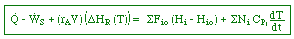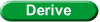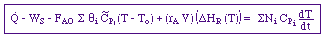Adiabatic batch reactor with no work: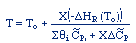#### Polymath

The following reaction occurs in a batch reactor: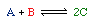1)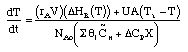2)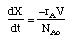3)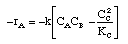4)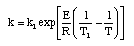5)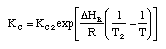6)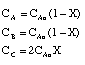7) Parameter Values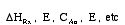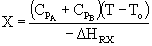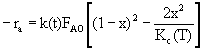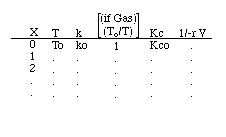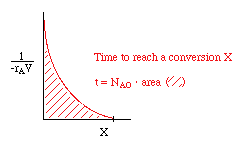or use one of the integration formulas, e.g.:, to find the reaction time, t. Even better, use Polymath.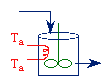Cooling: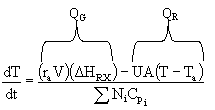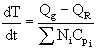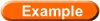Batch Reactor with External HeatingText Example 9-2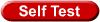Questions related to Problem 9-2

 Control of Chemical Reactors top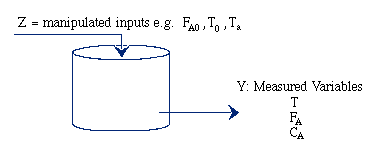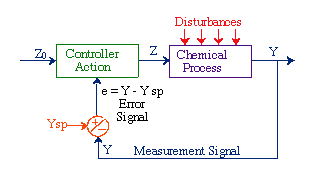Unsteady State CSTR: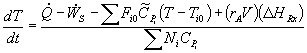For a batch reactor, FAO = 0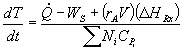#### Integral Control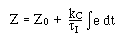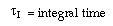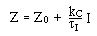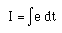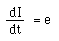For the reaction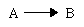in a CSTR: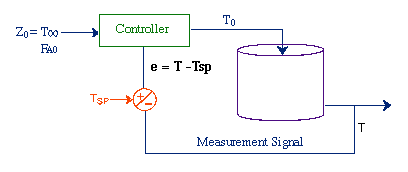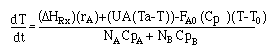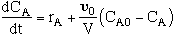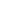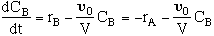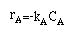#### Proportional Control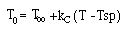#### Integral Controller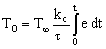#### Proportional-Integral Control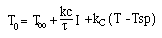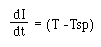Linearized Stability Theory top
 Energy Balance (Applied to a CSTR) 1.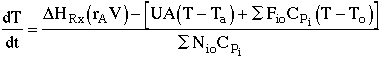2.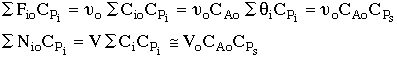3.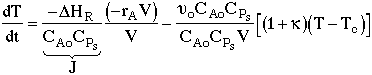4.CSTR Mole Balance 5.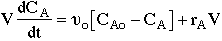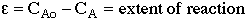6.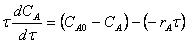Manipulating the Energy and Mole Balances Let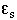and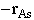signify steady state values. Then at steady state: 7.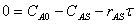Adding equations (6) and (7): 8.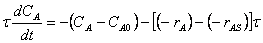Linearizing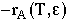by expanding it in a Taylor Series: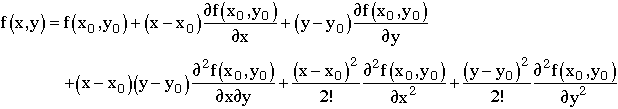To obtain: 9.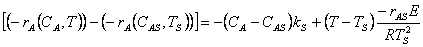10. Let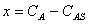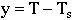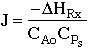11.12.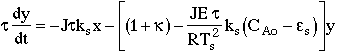13.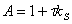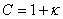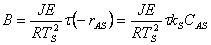Using these substitutions, we can arrive at the following equations that describe the behavior of temperature and concentration, when the steady state conditions are perturbed in a CSTR: 14.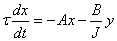15.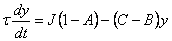16.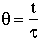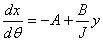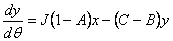17.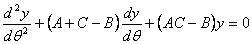18.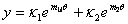19.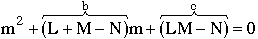20.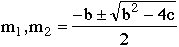21.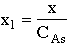22.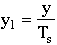Predicting the Behavior of a CSTR using LST top

At time t = 0, y1 = y10, where: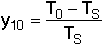Making use of Equation 20we notice that for the case of b2 = 4c:

if b < 0 ,   then the amplitude (i.e., T - TS) will increase

if b > 0 ,   then the amplitude (i.e., T - TS) will decrease

and that:

if b2 > 4c ,   then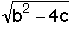is real (i.e., non-oscillatory behavior)

if b2 < 4c ,   thenis imaginary (i.e., oscillatory behavior)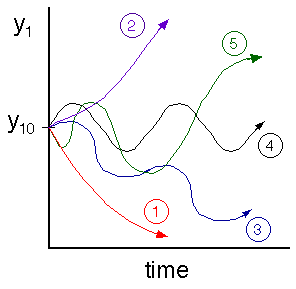Critically damped: b is positive andis real Unstable growth: b is negative andis real Oscillatory and damped: b is positive andis imaginary Oscillatory: b is zero andis imaginary Unstable growth oscillation: b is negative andis imaginary Reference: R. Aris, Elementary Chemical Reactor Analysis, Prentice Hall, New Jersey, (1969).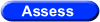Object Assessment of Chapter 9

* All chapter references are for the 4th Edition of the text Elements of Chemical Reaction Engineering .